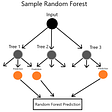# Applying Supervised Learning for Sports Betting

“Supervised Learning” refers to Machine Learning algorithms that learn how to predict an outcome from past knowledge. For instance if we want to train a model to predict the outcome of a game, we may feed it with player stats for the last 1000 games, telling it which team won each game. The model then “learns” how the player stats are related to the outcome of the game, and forms a relationship between the variables and the outcome. Once the model has learnt these relationships, we can give it player stats for upcoming games, and it will predict the outcome.

However, for us to use the model to run a profitable sports betting strategy, we need to do more than just predict the outcome- the model that predicts the outcomes most accurately is not necessarily the one that is the most profitable. In this article, I explore why that is, and how we should evaluate models instead.

1. Data

We will be working with the UFC Dataset. This contains a lot of information for every UFC fight since 2010 — including betting odds. The data is available here, along with a description of what each field represents.

A quick note on American Betting Odds
The odds in the dataset are expressed as American Betting Odds. The odds for the fighter that is the favourite is expressed as a negative amount. This represents the amount you would have to bet to earn \$100. The odds for the underdog are expressed as a positive amount, and this represents how much you would win if you bet \$100.

For instance, for the recent fight between Khabib Nurmagomedov and Justin Gaethje, the odds were:

Khabib = -315
Justin = 255

This means that to earn a profit of \$100, I would have to place a bet of \$315 on Khabib. On the other hand, if I placed a bet of \$100 on Justin, I would stand to earn a profit of \$255 if he won.
We can compute the profit we would earn if we bet a given amount on each fighter as:

2. Favourites for a reason!

In our dataset, the favourites win the fight ~65% of the time. What if we implemented a simple betting strategy, always betting on the favourites? We would win the bet more often than we would lose, but would this result in a profit? Let’s see what happens:

We start with a capital of \$5000, and place a bet of \$100 on the favourites in each fight.

The outcome of this betting strategy is:

While we were correct 65% of the time, we still ended up losing \$16,333, i.e. we went bankrupt! That is because betting on the favourites meant that on average we earned only \$47 every time we won the bet, but lost \$100 every time we were wrong.

It is therefore clear that simply choosing a model with the highest prediction accuracy is potentially ruinous. We need to choose a model based on the profit it generates, not purely it’s prediction accuracy.

3. Using Supervised Learning algorithms

I have used the following algorithms as potential betting models:

a. Logistic Regression
b. Decision Tree Classifier
c. Random Forest Classifier
e. Neural networks (Multi Layered Perceptron Classifier)

A discussion of technical details about each of these algorithms, such as how they are calibrated and the parameters they take is beyond the scope of this article. The Scikit Learn implementation of each of these algorithms in Python is used for model calibration. More details can be found here.

First we need to decide which player statistics are relevant and should be passed to the models. Then we need to create a split our data into 2 samples- the “training” sample which represents the past experience that we will feed into our model for it to learn, and the “testing” sample, which we will pass to our model to generate predictions (i.e. the model will not know the outcome of these fights- it will have to apply what it is has learnt on the training sample to predict the outcomes). I have used 80% of the data for training and 20% for testing.

We will also scale the features using the StandardScaler from Scikit Learn. This is to reduce the impact that outliers have on our model.

The model will return the predicted probability of each fighter winning the fight. Our betting strategy is then simple: we bet on the fighter the model thinks is most likely to win. However, we do not need to bet on every fight: we can decide to only bet if the model has at least a certain degree of confidence in the prediction. For instance, we will only bet if the predicted probability of winning is at least 60%.

Let’s set up the data and write a function that does all the hard work for us- including splitting the data between the test and training samples, scaling the features, training the model, generating predictions, and implementing our betting strategy. We will set up the function to tell us:

• How accurate the model is in terms of predicting the outcomes in the testing dataset
• The number of fights we placed a bet on (where the model was sufficiently confident in it’s prediction)
• How many bets we won
• The profit earned on average for each bet we won
• The total profit earned by implementing the strategy over the entire testing sample

4. Results

We can see that all our models have similar prediction accuracies- approximately 65–70%. However, 4 out of 5 of these models actually lose money. This includes the Gradient Boosting algorithm, which has the highest prediction accuracy of all the models considered.

The only model that earns a profit is the Neural Network, which is the model with the highest average win size- i.e. it earns the most money on average for each bet that it wins.

Incidentally, if we had run the same Neural Network model without scaling our features (our function allows us to simply state scale=False to skip this step), we would have actually lost money (see below)! This underscores the importance of scaling our data before training our models.

Conclusion
Using a model for sports betting is about more than just predicting the correct outcome. The model’s performance as a betting strategy depends not only on how many fights is correctly predicts, but which fights it predicts correctly i.e. how favourable the odds are for the fights it correctly predicts.

--

--

--

Data Science and Quantitative Finance

Love podcasts or audiobooks? Learn on the go with our new app.

## Milvus In Action: Chemical Structure Similarity Search## What is a Vector Database?## ROC curves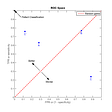## Multi-Layer Perceptrons (MLPs)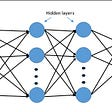## Advancing Natural Language Processing (NLP) for Enterprise Domains## Predicting Captchas By using Machine Learning And Segmentation- Make An Optical Character…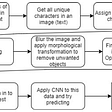## Exploiting hidden vectors in Long short-term memory (LSTM) networks for stock trading prediction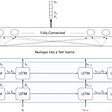Data Science and Quantitative Finance

## How to parallelize Naive Bayes classification?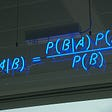## The Art of Choice: Supervised Machine Learning Work-flow for Intermediate Beginners## How to detect noisy data / outliers?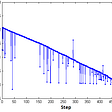## Imagine Recognition Through Supervised Learning in Python